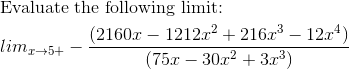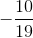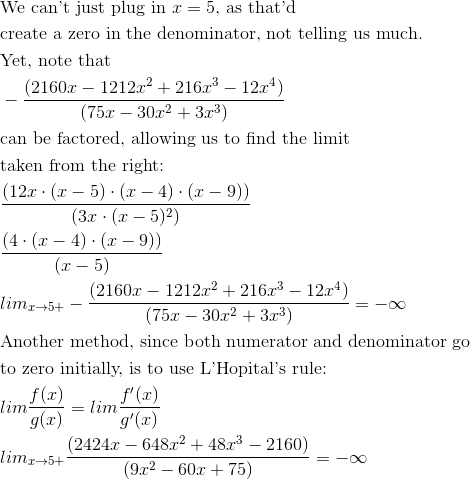Calculus 3 : Partial Derivatives

Example Questions

Example Question #81 : Limits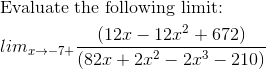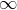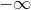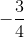Explanation: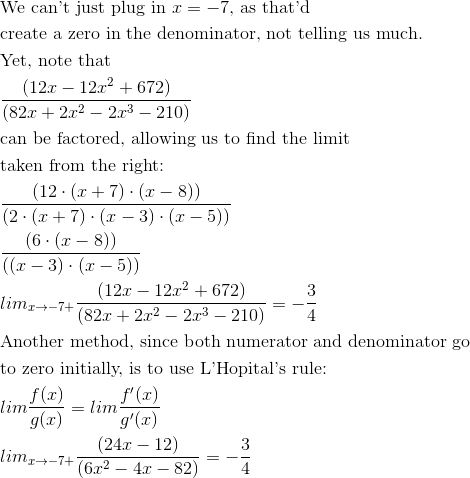Example Question #1041 : Calculus 3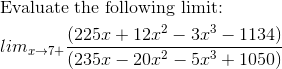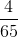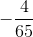Explanation: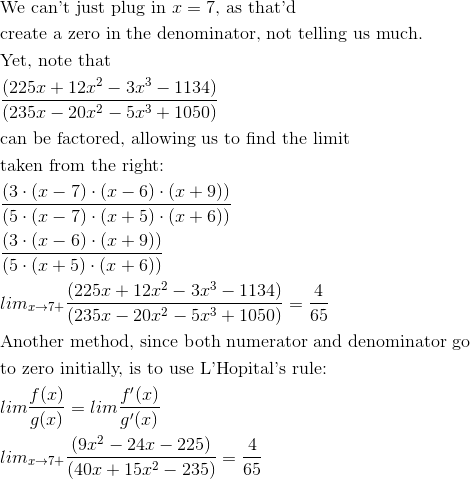Example Question #1042 : Calculus 3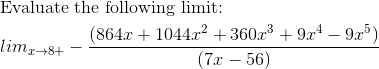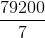Explanation: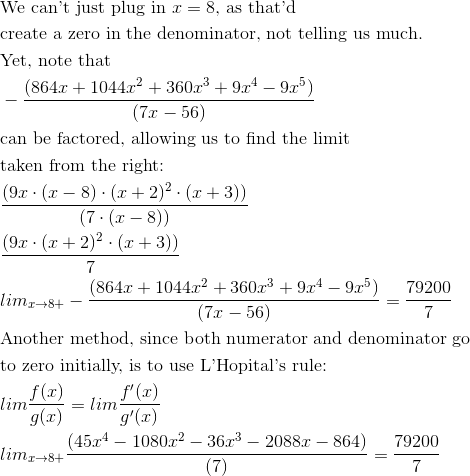Example Question #1043 : Calculus 3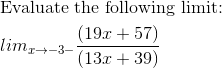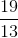Explanation: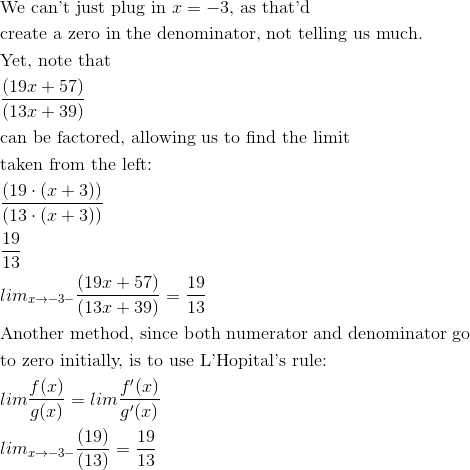Example Question #1044 : Calculus 3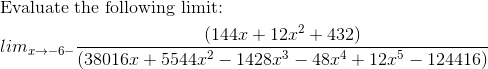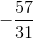Explanation: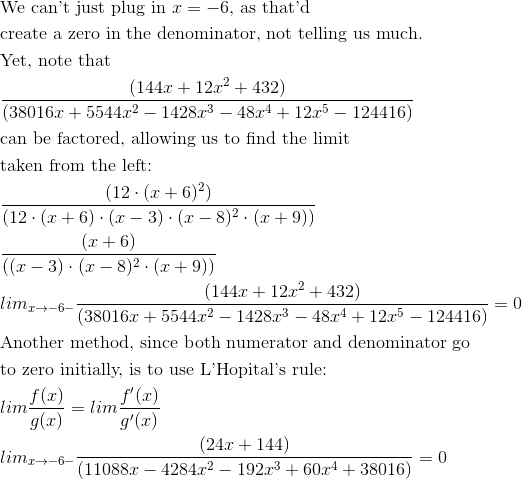Example Question #1045 : Calculus 3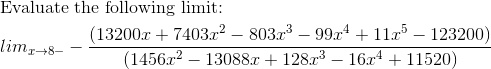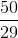Explanation: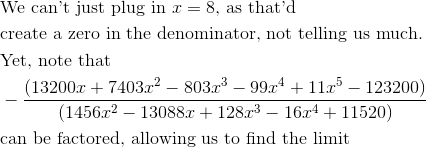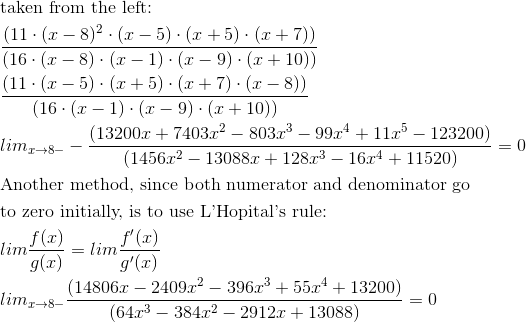Example Question #1046 : Calculus 3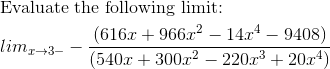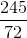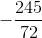Explanation: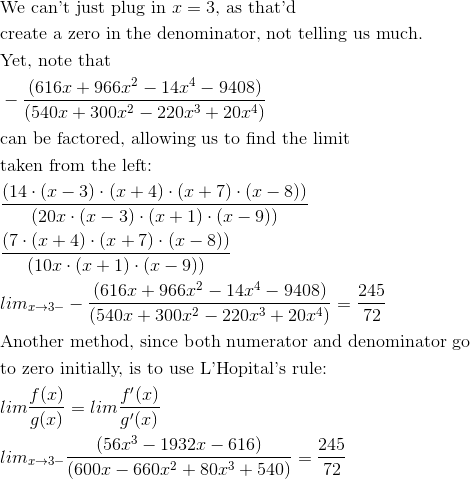Example Question #1047 : Calculus 3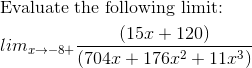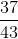Explanation: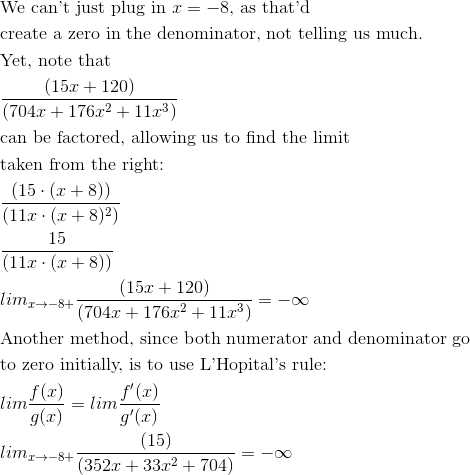Example Question #1048 : Calculus 3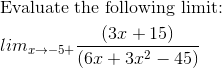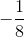Explanation: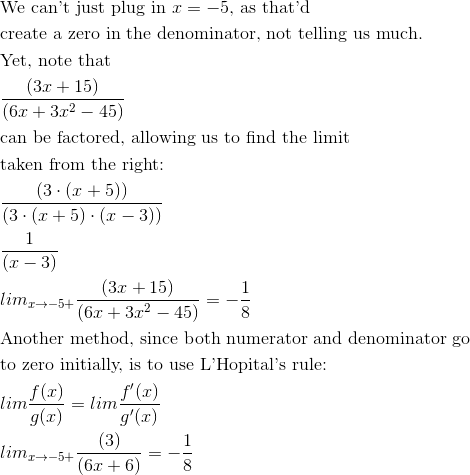Example Question #1049 : Calculus 3